Next: Projective Bases for the Up: Some Standard Cross Ratios Previous: Cross Ratios and Projective

## Cross Ratios of Pencils of Lines

Let U and V be two lines in the projective plane, defined by their dual coordinate vectors. Consider a set of linesdefined by: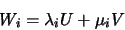with the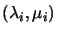defined up to scale as usual.belongs to the pencil of lines through the intersection of U and V (c.f. section 2.2.1), so each Wi passes through this point. As in section 3.1.1, the cross ratio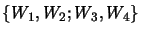is defined to be the cross ratio of the four homogeneous coordinate pairs. Dually to points, we have:

Theorem: The cross ratio of any four lines of a pencil is invariant under collineations.

Cross ratios of collinear points and coincident lines are linked as follows:

Theorem: The cross ratio of four lines of a pencil equals the cross ratio of their points of intersection with an arbitrary fifth line transversal to the pencil (i.e. not through the pencil's centre) -- see fig. 3.1.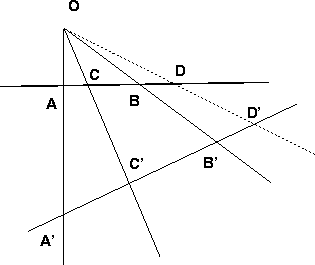In fact, we already know that the cross ratios of the intersection points must be the same for any two transversal lines, since the lines correspond bijectively to one another under a central projection, which is a collineation.

The simplest way to establish the result is to recall the line intersection formulae of section 2.2.1. If U and Vare the basis 3-vectors for the line pencil, and L is the transversal line, the intersection points of L with U, V and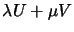are respectively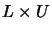,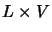and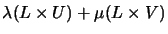. In other words, the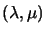coordinates of a line in the basis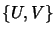are the same as thecoordinates of its intersection with L in the basis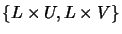. Hence, the two cross ratios are the same.

To establish the result, we will consider a particular transversal line: the line at infinity. Let ax0 + bx1 + cx2 = 0 be the equation defining U and dx0 + ex1 + fx2 = 0 the one corresponding to V. Therefore the one associated tois: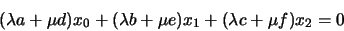This line intersect the line at infinity at a point with coordinates: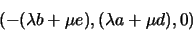If we consider two reference points M and N being the intersection with line U and V, i.e. the points with coordinates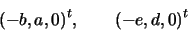the generic point can therefore be described as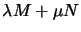. Therefore the intersection of line Wi with the line at infinity has the coordinateson the line [M, N], and therefore from the definition their cross ratios coincide.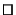Another way to prove the result is to show that cross ratios of lengths along the transversal line can be replaced by cross ratios of angle sines, and hence are independent of the transversal line chosen (c.f fig. 3.1):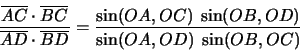(3.3)

However, this is quite a painful way to compute a cross ratio. A more elegant method uses determinants:

Theorem (Möbius): Let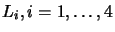be any four lines intersecting in O, and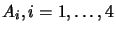be any four points respectively on these lines, then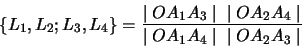(3.4)

where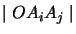denotes the determinant of the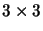matrix whose columns are the homogeneous coordinate vectors of points O, Ai and Aj.

This 19th century result extends gracefully to higher dimensions. To prove it, let (a,b, 1), (x,y, 1) and (u,v, 1) be the normalized affine coordinate vectors of O, Ai and Aj. Then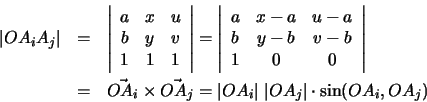The vector's lengths cancel out of the cross ratio of these terms, and if the coordinate vectors do not have affine normalization, the scale differences cancel out too.Next: Projective Bases for the Up: Some Standard Cross Ratios Previous: Cross Ratios and Projective
Bill Triggs
1998-11-13